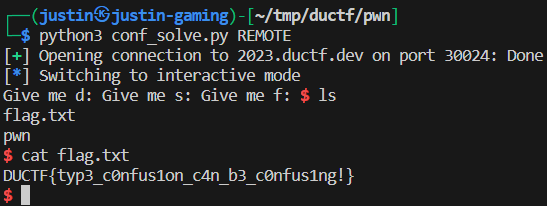# DUCTF 2023 - Confusing

## Writeup

Okay, this challenge was aptly named. The worst part about it wasn’t matching inputs to types, it was figuring out how the heck floats work in C (you don’t want to know). But let’s dive right in (file 1, file 2).

There are 4 stages to pop a shell, and each involves setting a different variable correctly. The type each variable is initialized as is different than what form our input comes in.

### d

The `d` variable is initialized as a signed short, which is just 2 bytes (16 bits) of data. The input is a long float, or double-precision floating point number, and the output needs to equal `13337` (`0x3419`). The fact that the input is a double-precision float instead of single-precision is important, as it changes how the number is stored. Floats are stored in a really funky way, with the 1st bit being positive/negative, the 2nd-9th bits being the exponent, and remaining bits being the mantissa. The number is then calculated as `sign * 2**exponent * mantissa`. This whole conversion process sucks, so I found a site online to convert the values between doubles and hex.

Since only 2 bytes of data can be stored but the `printf` statement takes in 8 bytes, the input will be truncated to only 2 bytes. This means that we only care about the last 4 hex digits in the input, which need to equal `0x3419`. We chose to use the complete hex value `0x3fc7ffffffff3419`, where you can see the last 4 digits are `13337` (the rest we’ll explain later). The converter turns this into the double `0.1874999999985512`.

### z

Before we get to the other 2 inputs, it’s important to note that there is no input for `z`, nor is it initialized to any value. How can we set it to our desired value? Well, even though the order of initializations in the source file is `d, f, s, z`, the `z` variable is actually placed right after the `d` variable. Remember how the input took 8 bytes (for a double-precision float), but the `d` variable can only store 2? Well, that means there’s 6 bytes of overflow that JUST HAPPEN to overflow the `z` variable :)

This means that out of the 8 bytes, the first 2 don’t matter, the middle 4 are `z`, and the last 2 are `d`. This why we chose `0x3fc7ffffffff3419`, since the middle 4 bytes are `0xffffffff`, which is the signed integer value for -1.

### s

Next we have `s`; this is initialized as 4 chars which must equal `FLAG`, and the input is a signed integer (also 4 bytes). This works out pretty nicely since the input is the same size as the storage. The characters `FLAG` are stored as hexadecimal `0x47414c46` (backwards), which is represented as `1195461702` as a decimal number.

### f

The last variable is `f`, stored as an 8-byte double-precision float that must equal `1.6180339887`, but the input is 8 characters. Using the same converter from the `s` variable, we found the hex value for `1.6180339887` is `0x3ff9e3779b9486e5`. Since many of these are non-printable characters, I converted my solution into a `pwntools` Python script that would do the inputs for me.

### SolveFlag: `DUCTF{typ3_c0nfus1on_c4n_b3_c0nfus1ng!}`